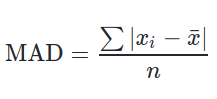# Python | Pandas Series.mad() to calculate Mean Absolute Deviation of a Series

Pandas provide a method to make Calculation of MAD (Mean Absolute Deviation) very easy. MAD is defined as average distance between each value and mean.

The formula used to calculate MAD is:Parameters:
axis: 0 or ‘index’ for row wise operation and 1 or ‘columns’ for column wise operation.
skipna: Includes NaN values too if False, Result will also be NaN even if a single Null value is included.
level: Defines level name or number in case of multilevel series.

Return Type: Float value

Example #1:
In this example, a Series is created from a Python List using Pandas .Series() method. The .mad() method is called on series with all default parameters.

 `# importing pandas module  ` `import` `pandas as pd  ` `   `  `# importing numpy module  ` `import` `numpy as np  ` `   `  `# creating list ` `list` `=``[``5``, ``12``, ``1``, ``0``, ``4``, ``22``, ``15``, ``3``, ``9``] ` ` `  `# creating series ` `series ``=` `pd.Series(``list``) ` ` `  `# calling .mad() method ` `result ``=` `series.mad() ` ` `  `# display ` `result `

Output:

`5.876543209876543`

Explanation:

Calculating Mean of series mean = (5+12+1+0+4+22+15+3+9) / 9 = 7.8888

MAD = | (5-7.88)+(12-7.88)+(1-7.88)+(0-7.88)+(4-7.88)+(22-7.88)+(15-7.88)+(3-7.88)+(9-7.88)) | / 9.00

MAD = (2.88 + 4.12 + 6.88 + 7.88 + 3.88 + 14.12 + 7.12 + 4.88 + 1.12) / 9.00

MAD = 5.8755 (More accurately = 5.876543209876543)

Whether you're preparing for your first job interview or aiming to upskill in this ever-evolving tech landscape, GeeksforGeeks Courses are your key to success. We provide top-quality content at affordable prices, all geared towards accelerating your growth in a time-bound manner. Join the millions we've already empowered, and we're here to do the same for you. Don't miss out - check it out now!

Previous
Next